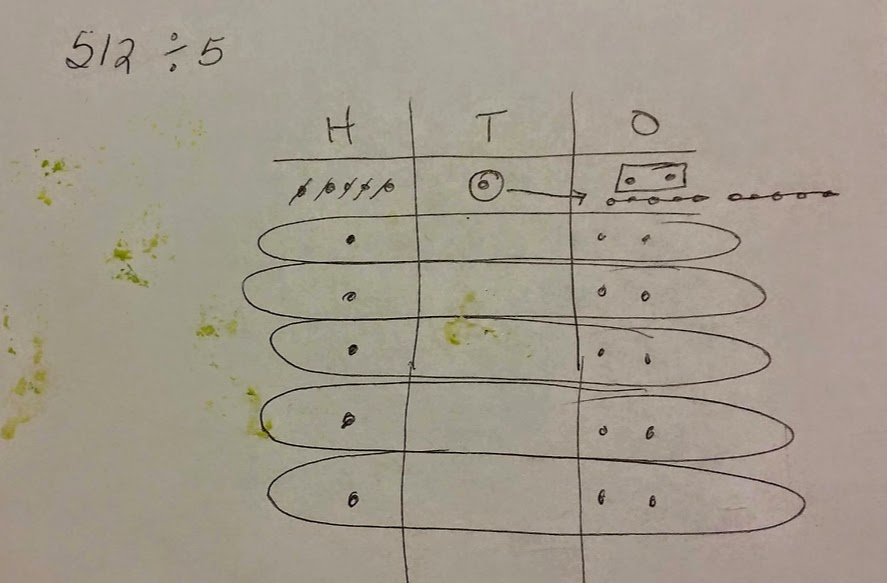# Place Value Chart to Divide Hundreds and Thousands

23 teachers like this lesson
Print Lesson

## Objective

SWBAT find whole number quotients and remainders using a place value chart.

#### Big Idea

In this lesson, students divide whole numbers by a one digit divisor, decomposing tens, hundreds, and thousands, to find a whole number quotients and remainders.

## Warm Up

5 minutes
Because basic facts are so important for my students to master, and more than half of my class does not have them mastered, I chose an oral division facts practice for this warm up.  I change the PowerPoint slides every 3 seconds. I encourage my students to yell their answers "loud and proud."

Being able to multiply and divide fluently within 100 is essential prior to fourth grade, and the basic facts of addition subtraction and multiplication/division are a critical baseline.  Students must be fluent in these skills in order to be successful with later work with fractions, decimals, ratios, and proportion. As students begin to learn math facts, their brains are focused on those basic computations, but as students become automatic with basic facts, their brains are then able to focus on other aspects of the task like the challenges of place value, decimals, or fractions. Being automatic with basic facts frees the brain to focus on other math processes. Committing basic math facts to memory speeds up math tasks.

## Concept development

40 minutes

This lesson is the third lesson in a series of lesson about using a place value chart to divide. To see the first lesson click here, and to see the second lesson, click here.

I chose to do this lesson series to give students a solid understanding of distribution as a way to divide. Before Common Core standards, teachers may have given a premature introduction to the standard division algorithm.  This does little to promote student understanding of the operation or of the meaning behind computational procedures. In classrooms where rote memorization of algorithmic steps is emphasized students often make computational errors without understanding why they are doing so.

I begin this lesson by showing a division problem on the board like 178 divided by 4.  I model the problem on the board, using a place value chart, as students model the problem in their math notebooks.  In today's lesson, students build and extend their learning from the previous lessons to solve division problems with dividends in the thousands and hundreds with remainders. Students will need to decompose thousands, hundreds, and tens to be successful with this lesson.  In the problem set students work on, I also include problems with a zero in the dividend and quotient,  and model with students how to record the quotient.

I give students 8 problems to work on during the class period.  Students that did not finish took it home as homework.  The problems students worked on in class and then took home were:

3248 / 3

1986 / 5

486 / 8

521 / 5

97 / 7

129 / 3

242 / 4

75 / 4